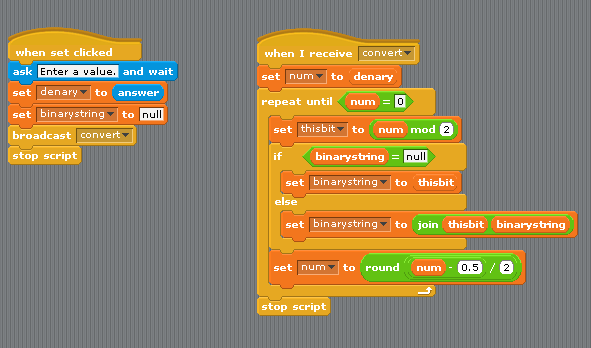# Scratch ProgrammingDenary To Binary Converter

## Introduction

This program is based on the following pseudocode description of the algorithm to convert a denary value into binary.

```Set num variable to a denary value to convert Set binarystring variable to 'null' Repeat Until num = 0    Set thisbit to num Mod 2    If binarystring = 'null then       Set binarystring to thisbit    Else       Set binarystring to Join (thisbit, binarystring)    End If    Set num to num ÷ 2, rounded down End Repeat```

## The Code